﻿ 一种基于稳态视觉诱发电位的脑控音乐播放系统 A Music Player System Based on Steady-State Visual Evoked Potential

Computer Science and Application
Vol. 09  No. 01 ( 2019 ), Article ID: 28437 , 11 pages
10.12677/CSA.2019.91006

A Music Player System Based on Steady-State Visual Evoked Potential

Ming Jin1, Weijie Yang2, Chen Zhao2

1School of Mathematics and Information Science, Guangxi University, Nanning Guangxi

2School of Computer and Information Engineering, Beijing Technology and Business University, Beijing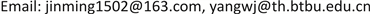Received: Dec. 24th, 2018; accepted: Jan. 7th, 2019; published: Jan. 14th, 2019ABSTRACT

Brain-computer Interfaces (BCI), is characterized by not relying on peripheral nerves, muscles, bones and other normal human body to control external devices directly controlled by the brain signal. BCI technology is developed rapidly, especially in the field of daily life, medical treatment, military affairs, entertainment, etc. This paper develops a music player system based on brain-computer interface. This system through the steady-state visual evoked potential (SSVEP) stimulates the brain to produce different brain electrical signals to drive the player to perform different music player instructions, realizes the remote transfer control instruction, and plays the music without key trigger. Apply of BCI technology in daily life helps people to realize the control mode “what you get is what you think” and provides a new way for the popularization of BCI.

Keywords:Brain-Computer Interface, Steady-State Visual Evoked Potential, Typical Correlation Analysis

1广西大学数学与信息科学学院，广西 南宁

2北京工商大学计算机与信息工程学院，北京Copyright © 2019 by authors and Hans Publishers Inc.1. 引言

2. 相关工作

1973年，脑–机接口的概念被Jacques Vidal等人首次提出  ，并将基于稳态视觉诱发电位控制脑–机接口系统的原理写入了个人学术论著里   。但是直到1999年第一届脑–机接口世界会议召开，才明确给出脑–机接口的概念与定义  ，脑–机接口技术才逐渐走进大众的视野。

2002年，第一个SSVEP-BCI系统由清华大学的高小榕教授等人提出   。该系统通过匹配分析EEG信号和变换到频域上的刺激频率，筛选出频域特征最相似的频率，从而确定EEG信号和其对应的刺激频率。2012年，Hochberg等人进行了一次植入式脑–机接口实验，实验对象是两名四肢瘫痪病人。在该实验中，这两名四肢瘫痪病人最终成功利用侵入式BCI系统自主操控机械臂完成三维抓握动作。基于此，我们似乎可以观测大脑的活动，预测人类的意图。然而当前所有种类的脑–机接口研究均不高效。以当前科学界对于脑–机接口技术的研究水平，最多只能做到让瘫痪病人通过BCI系统来操控机械臂完成一些简单的动作，且动作并不会和想象的一样规范、流畅  。这与科学界的所期望的“所想及所得”的目标相比，还有很大差距。

3. 稳态视觉诱发电位(SSVEP)信号采集

3.1. SSVEP概述

SSVEP的基本原理是给人类视网膜一个固定频率的闪烁刺激，从而诱发其大脑皮层枕叶部位产生一定的响应，从而得到不同的脑电信号。因为SSVEP的信号产生时间更快，传导速率也较高，所以相比于其他形式的脑-机接口系统，基于SSVEP的BCI系统具有更高的精度和更高的信息传输速率的优点。此外，SSVEP还是唯一的一种无需对被试者提前进行训练的脑–机接口范式，而且SSVEP范式中需要的EEG通道更少。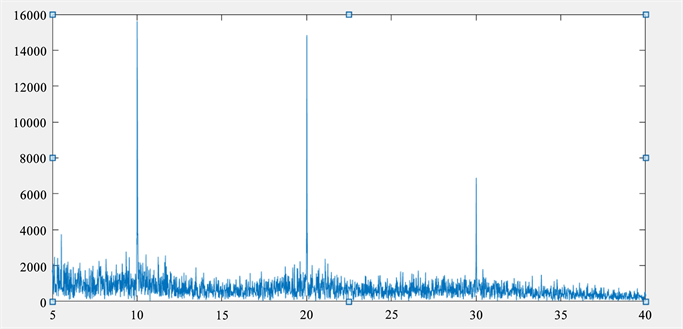Figure 1. FFT results of SSVEP signals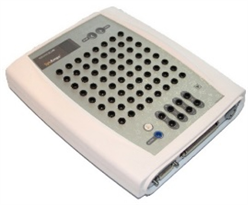Figure 2. SynAmps 2 EEG amplifier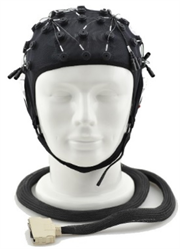Figure 3. NeuroScan electrode cap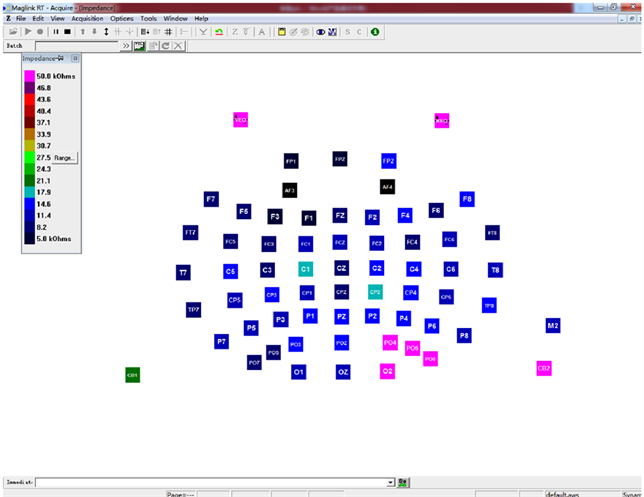Figure 4. Impedance interface of software SCAN

3.2. 离线SSVEP视觉刺激界面设计与实现

SSVEP信号是一种频率特异性的信号，因此，在频域提取特征也是一种有效的方式。所以在将微弱的脑电数据量化后，我们对采集到的特征信号进行FFT处理。则在SSVEP信号的频谱图上，刺激频率及其倍频谐波处信号幅值会发生显著的增加，该处会有明显的尖峰出现。这个规律可以作为后续脑电信号分类的特征。

A被试者的8 Hz SSVEP信号并不理想，该频率下有较大的噪声干扰，特征频率没有明显的区分度，所有的频率都混叠在一起，无法通过滤波等方法确认出特征频率。因此该频率应在A被试者的刺激信号中首先排除。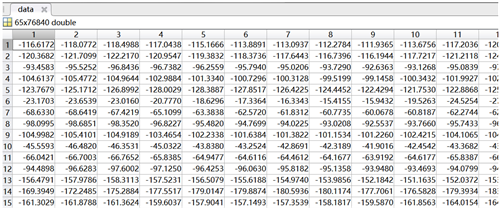Figure 5. EEG data processed by EEG-lab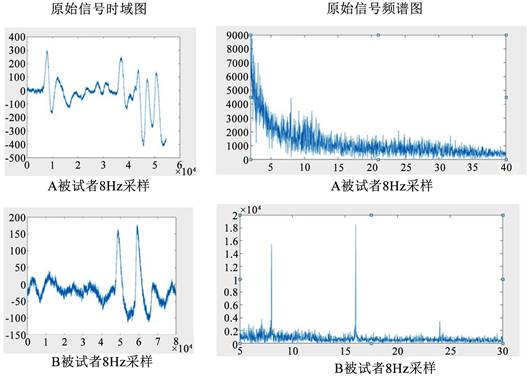Figure 6. Sensitive frequency time domain and spectrum diagram

4. 脑电信号处理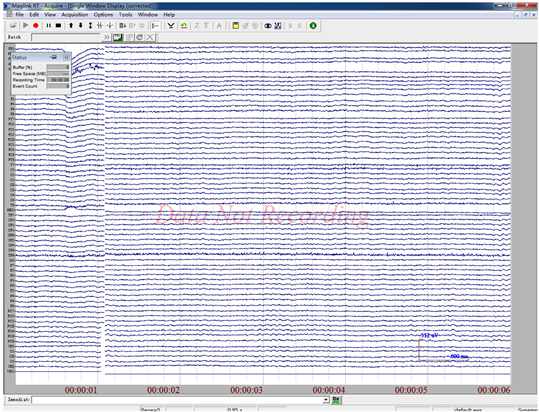Figure 7. EEG real-time acquisition page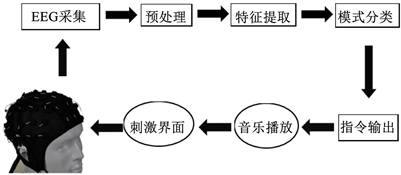Figure 8. Processing of EEG signals

1) 滤波：通过带通滤波或独立分量分析的方法对初始脑电信号分别进行基于频率和空间分布滤波，从而提高脑电信号的信噪比；

2) 下采样：由于采集到的原始脑电信号包含很多无用的信息，且其数据精度远远超出实际需求，因此需要对初始数据进行降维处理，以减少后续的数据处理量；

3) 去除伪迹：去除眼电、心电、肌电等伪迹或外界噪声干扰。

$U={W}_{x}X=\underset{i=1}{\overset{m}{\sum }}{W}_{xi}{X}_{i}$ (1)

$V={W}_{y}Y=\underset{j=1}{\overset{m}{\sum }}{W}_{yj}{Y}_{j}$ (2)

X和Y的线性组合叫做典型变量。CCA的目的就是求出能够最大化U和V之间的相关系数 $\rho \left(U,V\right)$ ，其中 $\rho \left(U,V\right)$ 为：

$\rho \left(U,V\right)=\frac{Cov\left(U,V\right)}{\sqrt{Var\left(U\right)Var\left(V\right)}}$ (3)

$Cov\left(U,V\right)={W}_{X}^{T}Cov\left(X,Y\right){W}_{Y}$ (4)

$Var\left(U\right)={W}_{X}^{T}Cov\left(X\right){W}_{X}$ (5)

$Var\left(V\right)={W}_{Y}^{T}Cov\left(Y\right){W}_{Y}$ (6)

$\mathrm{max}\left({W}_{x},{W}_{y}\right)\rho \left(U,V\right)=\frac{{W}_{X}^{T}Cov\left(X,Y\right){W}_{Y}}{\sqrt{{W}_{X}^{T}Cov\left(X\right){W}_{X}{W}_{Y}^{T}Cov\left(Y\right){W}_{Y}}}$ (7)

${y}_{i}\left(t\right)=\left(\begin{array}{l}{y}_{1}\left(t\right)\hfill \\ {y}_{2}\left(t\right)\hfill \\ {y}_{3}\left(t\right)\hfill \\ {y}_{4}\left(t\right)\hfill \\ {y}_{5}\left(t\right)\hfill \\ {y}_{6}\left(t\right)\hfill \end{array}\right)=\left(\begin{array}{l}\mathrm{sin}\left(2\text{π}{f}_{i}t\right)\hfill \\ \mathrm{cos}\left(2\text{π}{f}_{i}t\right)\hfill \\ \mathrm{sin}\left(4\text{π}{f}_{i}t\right)\hfill \\ \mathrm{cos}\left(4\text{π}{f}_{i}t\right)\hfill \\ \mathrm{sin}\left(6\text{π}{f}_{i}t\right)\hfill \\ \mathrm{cos}\left(6\text{π}{f}_{i}t\right)\hfill \end{array}\right),t=\frac{1}{s},\frac{2}{s},\cdots ,\frac{T}{s}$ (8)

${f}_{t\mathrm{arg}et}=\mathrm{max}\left(k\right){\rho }_{k},k=1,2\cdots N$ (9)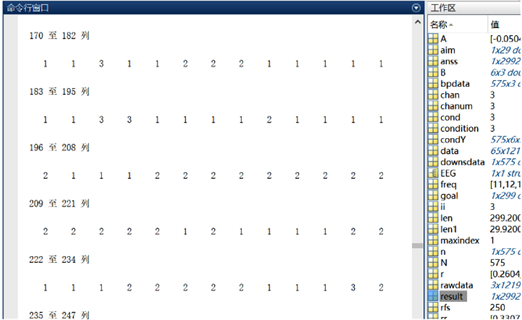Figure 9. Results of CCA

5. 基于BCI的音乐播放系统搭建与测试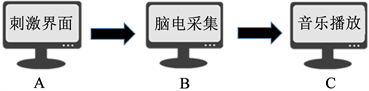Figure 10. Architecture of BCI music player system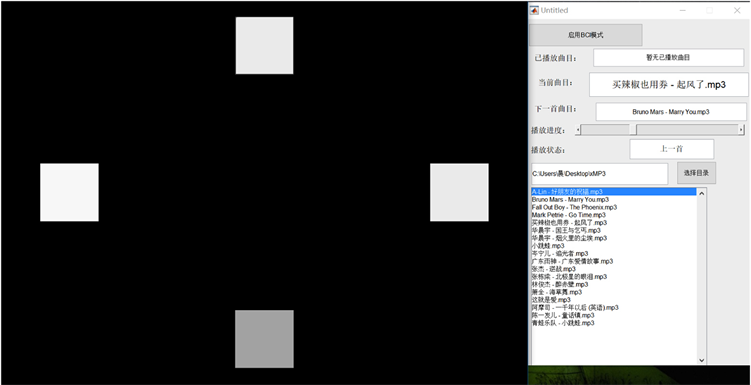Figure 11. Integrated interface of stimulation and music playing

6. 总结

A Music Player System Based on Steady-State Visual Evoked Potential[J]. 计算机科学与应用, 2019, 09(01): 46-56. https://doi.org/10.12677/CSA.2019.91006

1. 1. Vidal, J.J. (1973) Toward Direct Brain-Computer Communication. Annual review of Biophysics and Bioengineering, 2, 157-180. https://doi.org/10.1146/annurev.bb.02.060173.001105

2. 2. 李琳. 基于稳态视觉诱发电位的脑-机接口系统设计及相关算法的研究[D]: [硕士学位论文]. 杭州: 浙江理工大学, 2016.

3. 3. Lafleur, K., Cassady, K., Doud, A., et al. (2013) Quadcopter Control in Three Dimensional Space Using a Noninvasive Motor Imagery-Based Brain-Computer Interface. Journal of Neural Engineering, 10, 46003. https://doi.org/10.1088/1741-2560/10/4/046003

4. 4. Fazli, S., Mehnert, J., Steinbrink, J., et al. (2012) Enhanced Performance by a Hybrid NIRS-EEG Brain Computer Interface. Neuroimage, 59, 519-529. https://doi.org/10.1016/j.neuroimage.2011.07.084

5. 5. Wolpaw, J.R., Birbaumer, N., Mcfarland, D.J., et al. (2002) Brain-Computer Interfaces for Communication and Control. Clinical Neurophysiology, 113, 767-791. https://doi.org/10.1016/S1388-2457(02)00057-3

6. 6. Cheng, M., Gao, X., Gao, S., et al. (2002) Design and Implementation of a Braincomputer Interface with High Transfer Rates. IEEE Transactions on Biomedical Engineering, 49, 1181-1186. https://doi.org/10.1109/TBME.2002.803536

7. 7. Gao, X., Xu, D., Cheng, M., et al. (2003) A BCI-Based Environmental Con-troller for the Motion-Disabled. IEEE Transactions on Neural Systems and Rehabilitation Engineering, 11, 137-140. https://doi.org/10.1109/TNSRE.2003.814449

8. 8. 郭光瑞. 基于视觉诱发脑电的脑-机接口研究与实现[D]: [硕士学位论文]. 天津: 天津理工大学, 2016.